Next: Linear algebra Up: ALIAS-C++ Previous: Analyzing univariate polynomials

• La page de J-P. Merlet
• La page Présentation de HEPHAISTOS
• La page "Présentation" de l'INRIA

Subsections

# Parametric polynomials and eigenvalues of parametric matrices

A parametric polynomial is a polynomial whose coefficients are functions of a set of parameters (in other words it is a set of polynomials). A typical parametric polynomial is obtained when calculating the characteristic polynomial of a parametric matrix.

In this chapter we propose some algorithms to deal with the real roots of a parametric polynomial and, in some cases, with the real part of these roots. If the considered polynomial is the characteristic polynomial of a matrix we may make use of the components of the matrix.

Some of these algorithms use a primary and secondary algorithms. The secondary algorithm uses also a list of boxes which is stored in the interval matrix BoxUP.

# Minimal and maximal real roots of a parametric polynomial

The purpose here is to determine the minimal and maximal values of the set of real roots of the the set of polynomials, when the parameters are bounded. There may be eventually also constraints on the parameters. The algorithm is implemented as:


int ALIAS_Min_Max_EigenValues(int Degree,
int Nb_Parameter,
INTERVAL_VECTOR (* TheCoeff)(INTERVAL_VECTOR &),
int Nb_Constraints,
INTEGER_VECTOR &Type_Eq,
int (* TheMatrix)(INTERVAL_VECTOR &, INTERVAL_MATRIX &),
int Has_Matrix,
INTERVAL_VECTOR (* IntervalFunction)(int,int,INTERVAL_VECTOR &),
INTERVAL & TheDomain,
INTERVAL_VECTOR & TheDomain_Parameter,
int Type,int Nb_Points,int Use_Solve,int rand,
int M,
double Accuracy_Variable,double Accuracy,double AccuracyM,
INTERVAL &Lowest_Root,INTERVAL &Highest_Root,
INTERVAL_MATRIX &Place,int  Stop,double *Seuil,
int (* Solve_Poly)(double *, int *,double *),
int (* Simp_Proc)(INTERVAL_VECTOR &))

where the arguments are:
• Degree: degree of the polynomial
• Nb_Parameter: number of parameters appearing in the coefficients
• TheCoeff: a procedure that take as input an interval vector and returns the interval value of the coefficients of the polynomial. The elements of the input interval vector are first a range for the unknown in the polynomial, then the ranges for the Nb_Parameter parameters
• Nb_Constraints: the number of constraints expression that constraints the parameters
• Type_Eq: type of the constraints expressions (0 for equality, -1 for inequality of type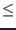0, 1 for inequality of type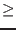0)
• TheMatrix: the polynomial may be the characteristic polynomial of a matrix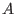. This argument is a procedure that takes as first argument the same interval vector as TheCoeff and returns in the second argument the interval component of. This procedure must return 1 if the components have been successfully calculated, -1 otherwise
• Has_Matrix: this flag must be set to 0 except if the polynomial is the the characteristic polynomial of a matrix in which case it must be set to 1 (2 if the matrix is symmetrical)
• IntervalFunction: a function which return the interval vector evaluation of the constraints and of the polynomial, the last component of the vector being the interval evaluation of the polynomial. This procedure must be written in ALIAS standard form, see note 2.3.4.3
• Has_Gradient: an array of integer that indicates if the derivatives of the expression are available. Only the first element is used for now with the following value:
• 0: no derivative available
• 1: only the derivatives of the constraints are available, not the one of the polynomial
• 2: all derivatives are available
• Gradient:a procedure which returns the Jacobian matrix of the expression for given values of the unknowns written in standard ALIAS form (see note 2.4.2.2)
• TheDomain: range for the polynomial unknown. This range must be large and is automatically adjusted during the calculation
• TheDomain_Parameter: the ranges for the parameters
• Type: 0 for finding only the minimum of the real root, 1 to find only the maximal root and 2 to find both
• Nb_Points: to estimate the minimal and maximal real root the algorithm compute the root of the polynomial at some given points for which the parameters have a fixed value. This value give the number of points where this procedure is used, it must be at least 1.
• Use_Solve: if this parameter is 1 or 3, then for each box we try to determine bounds for the real roots using algebraic geometry. If it is 2 or 3 then for each box we solve numerically the polynomial for some specific values of the parameters to update the minimum and maximum. If the value is 0 or 1 we will assume that a root of a polynomial is obtained when the width of the box is lower than Accuracy_Variable and the width of the evaluation of the polynomial is lower than Accuracy. If the confidence in the routine that solve numerically a polynomial is low the best choice is 1 otherwise the best choice is 3
• rand: every rand iteration the algorithm will consider that the current box is the one in the list that has the largest width. Such random permutation may allow to determine the minimal and maximal real root more quickly. This number must be neither too low (otherwise the maximal memory available may be exceeded) nor too large (otherwise the algorithm may focus on some part of the search space while the optimum is located in another part). A good compromise is 100.
• M: the maximum number of boxes which may be stored. See the note 2.3.4.5
• accuracy_Variable: the maximal width of the range of the polynomial unknown to be a solution, see the note 2.3.4.6
• Accuracy: the maximal width of the polynomial evaluation for a solution, see the note 2.3.4.6
• AccuracyM: the accuracy with which the optimum is determined. The absolute value of the difference between the real optimum and the calculated one should not exceed this value
• Lowest_Root, Highest_Root: point interval giving the minimal and maximal real root
• Place: the value of the parameters for which the optimum is obtained: the first line is for the minimum and the second line for the maximum
• Stop, Seuil: if Stop is set to 1
• when looking for a minimum only the procedure exit as soon as a minimum lower than Seuil has been found
• when looking for a maximum only the procedure exit as soon as a maximum greater than Seuil has been found
• when looking both for a minimum and a maximum the procedure exit as a minimum lower than Seuil OR a maximum greater than Seuil has been found
If Stop is set to 2 when looking both for a minimum and a maximum the procedure exit as a minimum lower than Seuil AND a maximum greater than Seuil has been found (otherwise as the same behavior than 1)
• Solve_Poly: a procedure that compute the real roots of a polynomial. It takes as argument the coefficients of the polynomial, a pointer to an integer that is initially the degree of the polynomial and the real roots are stored in the last argument. This procedure returns the number of real roots or -1 if the computation has failed. ALIAS provides as possible procedure ALIAS_Solve_Poly.
• Simp_Proc: a user-supplied procedure that take as input the current box and may proceed to some reduction of the width of the box or even determine that there is no solution for this box, in which case it should return -1.
The confidence in this procedure is at the same level than the confidence in the numerical algorithm that solve a polynomial.

The return code is:

• 1: algorithm has succeeded
• 0: result is not guaranteed
• -1: algorithm has failed, not enough memory
• -2: largest root of the polynomial lower than the given lower bound
• -3: smallest root of the polynomial larger than the given upper bound
• -4: error in the type of the equations
• -5: error, more than one function to optimize
• -100: in the mixed bisection mode the number of variables that will be bisected is larger than the number of unknowns
• -150: ALIAS_Delta3B or ALIAS_Max3B have not the right dimension Nb_Parameter+1
• -200: one of the value of ALIAS_Delta3B or ALIAS_Max3B is negative or 0
• -300: one of the value of ALIAS_SubEq3B is not 0 or 1
• -1000: Single_Bisection has an incorrect value
• -1500: Degree is lower than 0
• -2000: Nb_Parameter is lower or equal to 0
• -3000: we use the full bisection mode and the problem has more than 10 unknowns
• -3500: Nb_Constraints is lower than 0
• -4000: Type not between 0 and 2
• -4500: Stop_First_Sol not between 0 and 2
• -5000: Use_Solve not between 0 and 4
• -6000: Place has not 2 rows or Nb_Parameter+1 columns
• -6500: the initial estimate have incompatible lower and upper bound

The possible bisection mode are:

• 1: if the polynomial parameter has a value that is better than the current optimum, then this variable is bisected otherwise mode 1 of section 2.4.1.3
• 2: mode 1 of section 2.4.1.3 if the gradient is not available
• 3,4: mode 1 of section 2.4.1.3
• 5: mode 5 of section 2.4.1.3
For mode 2 if the gradient is available and if the polynomial parameter has a value that is better than the current optimum, then this variable is bisected otherwise we use the smear function to determine the bisected variable.

# Possible parameters values for a given range on the real roots

It may be of interest to determine what may be the possible values of the parameters of a parametric polynomial such that the real roots of the corresponding polynomial are all enclosed in a given interval. ALIAS provides two routines for that purpose.

## Approximation of the set of solutions

Let consider the parameters space i.e. a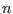dimensional space where each of the dimension corresponds to one of theparameters. A point in this space corresponds to a unique value for all the parameters and therefore to a specific polynomial. In the parameters space there are possibly a set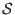of regions such that for any point in the region(s) the corresponding polynomial has all its root within the given interval. The purpose of the following procedure is to determine an approximation of. This approximation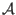will be constituted of a set ofdimensional boxes which are guaranteed to be included inand that will be written in a file. During the calculation the boxes whose width is lower than a given threshold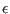and for which the algorithm has been unable to determine if they are fully enclosed inwill be neglected. A possible index for measuring the quality of the approximationis the ratio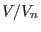between the total volume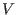of the boxes written into the file over the total volume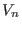of the boxes that have been neglected as the volume ofis lower or equal to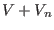.

The procedure is:


int ALIAS_Min_Max_EigenValues_Area(int Degree,int Nb_Parameter,
int Has_Interval,
INTERVAL_VECTOR (* TheCoeff)(INTERVAL_VECTOR &),
INTERVAL_VECTOR (* TheCoeffCentered)(INTERVAL_VECTOR &,double),
int Nb_Constraints,INTEGER_VECTOR &Type_Eq,
int (* TheMatrix)(INTERVAL_VECTOR &, INTERVAL_MATRIX &),
int Has_Matrix,
INTERVAL_VECTOR (* IntervalFunction)(int,int,INTERVAL_VECTOR &),
INTERVAL & TheDomain,INTERVAL_VECTOR & TheDomain_Parameter,
int Nb_Points,int Use_Solve,int rand,int Strong,int Iteration,
double Accuracy_Variable,double Accuracy,double AccuracyM,double AccuracyB,
double *Volume_Result,double *Volume_Neglected,double Seuil,
char *FileName,int Has_Input,char *File_Input,
int (* Solve_Poly)(double *, int *,double *),int RealRoot,
INTERVAL_VECTOR (* Evaluate_Complex)(int,int,INTERVAL_VECTOR &),
int (* Simp_Proc)(INTERVAL_VECTOR &))

where the arguments are similar to the one of the previous procedure except for:
• TheCoeffCentered: a procedure that returns the coefficients in x of the polynomial P(x+a). Takes as argument the parameters vector and a
• AccuracyB: the thresholdfor the maximal width of the neglected boxes
• Volume_Result: the total volume of the boxes that have been determined to be enclosed in the regions
• Volume_Neglected: the total volume of the boxes that have been neglected
• Seuil: the interval [Seuil, Seuil] defines the allowed range for the real roots of the polynomial
• FileName: the name of the file in which will be written the boxes that are included in the regions
• Has_Input, File_Input: the purpose of these variables is to allow an incremental improvement of the approximation. Indeed after a first run with a giventhe quality index may be not satisfactory. It is possible to improve it by decreasing the value offor a second run but it means that the boxes that have been determined to be enclosed in the region during the first run will be considered again, thereby leading to a loss of efficiency. These arguments allow a better control. During the first run if Has_Input has been set to 1 the neglected boxes will be stored in the file File_Input. During the second run (and the subsequent run if needed) Has_Input will be set to 2 and the set of boxes to be considered by the algorithm will be read from the file File_Input. During this type of run the neglected boxes will still be written in the file, allowing another run of the algorithm if needed. Hence the total volume of the boxes enclosed in the region will be the sum of the Volume_Result while the total volume of the neglected boxes will be the obtained during the last run of the algorithm. If Has_Input is set to 3 the neglected boxes will not be saved in a file.
• Strong: if 1 we use a secondary algorithm to determine if for a given box all the polynomial roots are in the range
• RealRoot: 0 if we are considering only real roots, 1 for the real part of the roots, 2 if we consider polynomial whose roots are all real and 3 if we consider polynomial with at least one real root
• Evaluate_Complex(i1,i2,X: let P be the polynomial and U=P(Seuil_First_Sol+I b), V=P(Seuil_First_Sol+I b). Let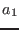be the real part of U,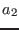the complex part of U,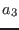the real part of V and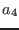the complex part of V. The procedure will return in its interval vector the value of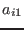to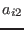. X is a Nb_Parameter+1 interval vector, the last one being the value of b

This procedure returns the number of boxes written in the result file or a negative number if the calculation has failed. The possible negative return code are:

• -2, -3: errors on the bounds for the roots
• -10: Iteration is lower than 10
• other values: the procedure that compute the minima and maximal real rots in a box has failed

## Largest square enclosed in the regions

This second procedure allows to compute the largest square (up to a pre-defined accuracy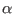) that is enclosed in the region. This largest box can clearly be obtained from the result of the previous algorithm but this weaker procedure will be faster.

The principle is to have a set of boxes whose first element is a range for the center of the box and second element is a possible value for the length of the half-edge of the square. The main algorithm will test if for a given box the center may be a candidate to be the center of a square of half-edge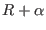(where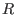is the current optimum) using some heuristics and if the answer is positive will compute the minimal and maximal root of the polynomials defined by this square using a secondary algorithm. If these values are compatible with the bound the current optimum will be updated.


int ALIAS_Geometry_Carre(int Degree,int Nb_Parameter,
INTERVAL_VECTOR (* TheCoeff)(INTERVAL_VECTOR &),
int Nb_Constraints,INTEGER_VECTOR &Type_Eq,INTEGER_VECTOR &Imperatif,
int (* TheMatrix)(INTERVAL_VECTOR &, INTERVAL_MATRIX &),
int Has_Matrix,
INTERVAL_VECTOR (* IntervalFunction)(int,int,INTERVAL_VECTOR &),
INTERVAL & TheDomain,INTERVAL_VECTOR & TheDomain_Parameter,
int Nb_Points,int Use_Solve,int rand,
int Iteration_Geometry,int Iteration_Polynom,
double Accuracy_Variable,double Accuracy,double Accuracy_Geometry,
double Accuracy_Polynom,INTERVAL_VECTOR &Solution,double *Seuil,
int (* Solve_Poly)(double *, int *,double *),
int (* Simp_Proc)(INTERVAL_VECTOR &),
int (* Simp_Proc_Pol)(INTERVAL_VECTOR &))

The arguments are the same than for the previous procedure except for:
• Imperatif: an array of integer. If there are constraints that are imperative, meaning that the polynomial cannot be evaluated if they are not satisfied (for example the constraints is that some term is positive as in the coefficients of the polynomial this term appears as a square root) you may set the corresponding integer to 1. If this array has dimension 0 all the constraint will supposed to be not imperative
• Iteration_Geometry: the number of boxes that may be used by the main algorithm
• Iteration_Polynom: the number of boxes that may be used by the secondary algorithm
• Accuracy_Geometry: the value of• Accuracy_Polynom: the accuracy used for the secondary algorithm. Note that this parameter play a role not only on the computation time but also on the bound for the root. Indeed the algorithm will verify that in the square there is no polynomial with a root lower than Seuil+Accuracy_Polynom or larger than Seuil-Accuracy_Polynom
• Solution: the point interval is the center of the largest square while the second is the value of the half-edge
• Simp_Proc: a simplification procedure used only in the main algorithm. The flag ALIAS_Simp_Main is set to 1 when this procedure is called right after the bisection process.
• Simp_Proc_Polynom: a simplification procedure used only in the secondary algorithm

This procedure will return:

• -1000: error in the Single_Bisection flag that should be between 0 and 5
• -4: error in Type_Eq
• -3: the lowest root of all the polynomials is greater than Seuil
• -2: the highest root of all the polynomials is lower than Seuil
• -1: the algorithm has failed
• 0: the result is not guaranteed
• 1:the result is guaranteed

During the calculation the flag ALIAS_Has_OptimumG will be set to 1 as soon as an optimum is found: the center of the current optimal geometry is the mid point of the interval vector ALIAS_Vector_OptimumG while is edge is in the interval ALIAS_OptimumG.

The secondary algorithm uses the flag Single_BisectionG and Reverse_StrorageG that play the same role than Single_Bisection and Reverse_Storage in the general solving algorithms (see sections 2.3.1.3 and 2.3.1.2).

# Condition number

The condition number of a polynomial may be defined either as the ratio lowest root over largest root or as the ratio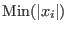over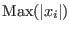where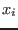are the roots of the polynomial. In the later case the condition number has a value between 0 and 1. The minimal and maximal values of the condition number of a parametric polynomial in both form may be calculated using the procedure:


int ALIAS_Min_Max_CN(int Degree,
int Nb_Parameter,INTERVAL_VECTOR (* TheCoeff)(INTERVAL_VECTOR &),
int Nb_Constraints,INTEGER_VECTOR &Type_Eq,
int (* TheMatrix)(INTERVAL_VECTOR &, INTERVAL_MATRIX &),
int Has_Matrix,
INTERVAL_VECTOR (* IntervalFunction)(int,int,INTERVAL_VECTOR &),
INTERVAL & TheDomain,INTERVAL_VECTOR & TheDomain_Parameter, int Type,
int Nb_Points,int Absolute,
int rand,int Iteration,
double Accuracy_Variable,double Accuracy,double AccuracyM,
INTERVAL &Lowest,INTERVAL &Highest,
INTERVAL_MATRIX &Place,int  Stop, double *Seuil,
int (* Solve_Poly)(double *, int *,double *),
int (* Simp_Proc)(INTERVAL_VECTOR &))

where the arguments are identical than for the previous procedure except for:
• Absolute: 0 if looking for the ratio minimal root over maximal root, 1 if looking for the ratio in absolute value
• AccuracyM: the accuracy with which the ratio will be computed
• Lowest, Highest: minimal and maximal value of the condition number
This procedure allows for the calculation of the minimal and maximal condition number of a matrix. Various bisection methods are available through the use of the integer Single_Bisection:
• 1: mode 1 of section 2.4.1.3
• 2: mode 6 of section 2.4.1.3 if the gradient is not available
• 3,4: mode 1 of section 2.4.1.3
• 5: mode 5 of section 2.4.1.3
A specific procedure is used when the gradient is available. If the interval for the root includes 0 we bisect it. Otherwise we use the smear function to decide which other variable should be bisected.

# Kharitonov polynomials

Kharitonov polynomials are special polynomials that have constant values for their coefficients and are associated to a parametric polynomial. It can be shown that if all the Kharitonov polynomials have the real parts of their roots of the same sign, then all the polynomials in the set will have the real part of their roots of the same sign. For a polynomial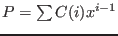the four Kharitonov polynomials are: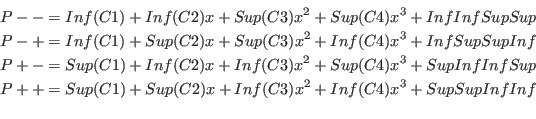## Implementation

The following procedure allows to determine if a polynomial has a real root within a given range:

int Kharitonov(int Degree,
INTERVAL_VECTOR (* TheCoeff)(double a,INTERVAL_VECTOR &),
int (* Solve_Poly)(double *, int *,double *),
INTERVAL_VECTOR &Input)

where:
• Degree: the degree of the polynomial
• TheCoeff: a procedure that allows to compute the interval coefficients of the polynomial at a point a (i.e. the coefficients of the polynomial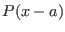)
• Solve_Poly: a procedure that compute the real part of the roots of a polynomial. It takes as argument the coefficients of the polynomial, a pointer to an integer that is initially the degree of the polynomial and the real roots are stored in the last argument. This procedure returns the number of roots or -1 if the computation has failed. ALIAS provides as possible procedure ALIAS_Solve_Poly_PR.
• Input: the range for the root
Note that there exists an ALIAS-Maple procedure that uses this procedure to generate a simplification procedure, see the ALIAS-Maple manual.

# Gerschgorin circles

## Mathematical background

Let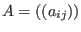and the Gerschgorin circles defined as the set of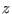such that: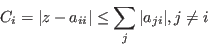The roots of the characteristic polynomial are enclosed in the union of the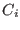. Furthermore if a circle has no intersection with the other circles, then this circle contains one root of the polynomial. This allow for a fast determination of bounds for the real roots of the polynomial.

## Implementation

It is possible to get these bounds by using the procedure:


int Gerschgorin(INTERVAL_MATRIX &A,int Size, int Type, INTERVAL &Bound)

where:
• A: the matrix
• Size: the dimension of the matrix
• Type: 0 if the matrix is not available, 2 if the matrix is symmetrical, 1 otherwise
• Bound: all the roots will be enclosed in this interval
the procedure returns 1 if it has been possible to compute Bound.

A more complete procedure allows to get all the Gerschgorin circles and eventually to adjust an interval that is supposed to contain the roots of the polynomial:


int Gerschgorin_Simplification(INTERVAL_MATRIX &A,int Size,int Type,
INTERVAL &Input,INTERVAL_VECTOR &Circle)

The arguments are the same than the previous procedure except for Input which is the interval supposed to contain roots of the polynomial and Circle which contain the projection of the Gerschgorin circle on the real axis. This procedure returns:
• -1: Input does not contain a root of the polynomial
• 0: no change in Input
•1: Input has been improved and the return value gives the number of distinct circles

# Cassini ovals

## Mathematical background

The Cassini ovals are another method to determine a bound for the eigenvalues of a matrix. Letand the Cassini ovals defined as the set ofsuch that: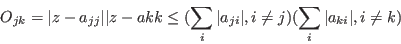Column based Cassini ovals may also be defined. The roots of the characteristic polynomial are enclosed in the union of the row-based and column-based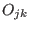. Although more complicate to calculate the bounds obtained with the Cassini ovals are usually tighter than the bounds obtained with the Gerschgorin circles.

## Implementation

The Cassini bounds may be obtained with the procedure:


int Cassini_Simplification(INTERVAL_MATRIX &A,int Size,
int Type_Matrix, INTERVAL &Input)

where
• A: the matrix
• Size: the dimension of the matrix
• Type: 0 if the matrix is not available, 2 if the matrix is symmetrical, 1 otherwise
• Input: an estimation of the bounds of the roots
The procedure will return -1 if there is no intersection between Input and the Cassini bounds, 1 if there Input has been improved, 0 otherwise.

This procedure may also be called with:


int Cassini_Simplification(int (* TheMatrix)(INTERVAL_VECTOR &, INTERVAL_MATRIX &),
INTERVAL_VECTOR &Param,int Size, int Type_Matrix, INTERVAL &Input)

where TheMatrix is a procedure that compute the elements of the matrix according to the interval value of the parameters Param.

# Routh

The Routh algorithm allows to calculate the number of roots with positive real part of a polynomial being given the coefficients of the polynomial. It is implemented as:


int Routh(int Degree,double *Coeff)

that returns the number of roots with positive real part or -1 if it has not been possible to compute the Routh table (because the first element of a row of the Routh table is close to 0).

A similar algorithm allows to deal with polynomial with interval coefficients:


INTERVAL Routh(int Degree,INTERVAL_VECTOR &Coeff)

This algorithm returns in its interval:
-1,-1
: the Routh table cannot be computed
a,a+1
: there is at least a roots with positive real part but the exact number of roots with positive real part cannot be calculated
a,a
: there is exactly a roots with positive real part
A similar procedure may be used when the coefficients are functions of parameters:

INTERVAL Routh(int Degree,INTERVAL_VECTOR (* TheCoeff)(int,int,INTERVAL_VECTOR &),
INTERVAL_VECTOR &Input)

where:
• TheCoeff: a procedure that allow to calculate the coefficients being given the range for the parameters. It returns a Degree+1 interval vector. The two first integers l1, l2 of the procedure allows one to specify which coefficients are calculated. For example if V=TheCoeff(1,5,Input), then V(1..5) will be the first 5 coefficients of the polynomial and if V=TheCoeff(1,Degree+1,Input), then all the coefficients of the polynomial will be available in V
• Input: the intervals for the parameter
If the derivatives of the coefficients with respect to the parameters are known an even better procedure will be:

INTERVAL Routh(int Degree,INTERVAL_VECTOR (*TheCoeff)(int,int,INTERVAL_VECTOR &),
INTERVAL_MATRIX (* TheCoeffG)(int,int,INTERVAL_VECTOR &),
INTERVAL_VECTOR &Input)

where TheCoeffG is a procedure that allow to compute the derivatives of the coefficients with respect to the parameters (see note 2.4.2.2). This procedure allows to a certain amount to take into account the dependency between the coefficients.

Note also that the procedure Routh of ALIAS-Maple allows an even better calculation of the Routh table when dealing with parametric polynomials as the elements of the Routh table are calculated symbolically.

# Weyl filter

## Mathematical background

Let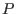be a polynomial and be maxroot the maximal modulus of the root of. Fromwe may derive a the unitary polynomial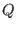such that the roots ofhave a modulus lower or equal to 1 and if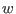is a root ofthen maxrootis a root of.

Let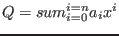which may also be written as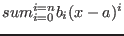where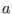is some fixed point.

Let a range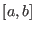for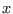and let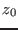be the mid point of the range. We consider the square in the complex plane centered atand whose edge length is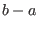. Let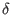be the length of the half-diagonal of this square. If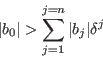then the polynomial has no root in the square . This procedure may be used for univariate polynomial, with polynomial with interval coefficients or with parametric poynomial. For example it is very efficient for solging the Wilkinson polynomial.

## Implementation

The Weyl filter is implemented to manage univariate polynomial (with numerical or interval coefficients) or parametric polynomial. A basic tool of this filter is


int Weyl_Filter_Utility(int Degree, double maxroot,
INTERVAL_VECTOR &b,INTERVAL_VECTOR &Input)

where Input(1) is the range for the polynom variable and b the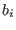coefficients. A more general implementation is

int Weyl_Filter(int Degree, INTERVAL_VECTOR &Coeff,double maxroot,
int (* GetB)(int,INTERVAL_VECTOR &,INTERVAL &,INTERVAL_VECTOR &),
INTERVAL_VECTOR &Input)

Here GetB is a procedure that calculates thecoefficients. Its arguments are the degree of the polynomial, its coefficients,and thecoefficients. It should return 1 if the calculation has been successfull, 0 otherwise. An example of such procedure is Derive_Polynomial_Expansion. Degree is the degree of the polynomial, Coeff its coefficients and Input(1) is the range for the polynomial unknown.

This procedure has several variants:


int Weyl_Filter(int Degree, INTERVAL_VECTOR &Coeff,double max_root,
INTERVAL_VECTOR &Input)

here the GetB procedure will be Derive_Polynomial_Expansion. In

int Weyl_Filter(int Degree, INTERVAL_VECTOR &Coeff,
INTERVAL_VECTOR &Input)

here the GetB procedure will be Derive_Polynomial_Expansion and maxroot will be computed by an ALIAS procedure. The same procedure will be used in

int Weyl_Filter(int Degree, INTERVAL_VECTOR &Coeff,
int (* GetB)(int,INTERVAL_VECTOR &,INTERVAL &,INTERVAL_VECTOR &),
INTERVAL_VECTOR &Input)


# Coefficient of the characteristic polynomial

In some cases it may be difficult to obtain the analytical form of the coefficients of the characteristic polynomial of a matrix. The following procedure allows to compute these coefficients even if the matrix is an interval matrix:


INTERVAL_VECTOR Coeff_CharPoly(int Size,INTERVAL_MATRIX &A)

where Size is the dimension of the matrix and A the matrix. This procedures returns the coefficients in an interval vector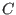and the polynomial is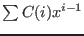.Next: Linear algebra Up: ALIAS-C++ Previous: Analyzing univariate polynomials